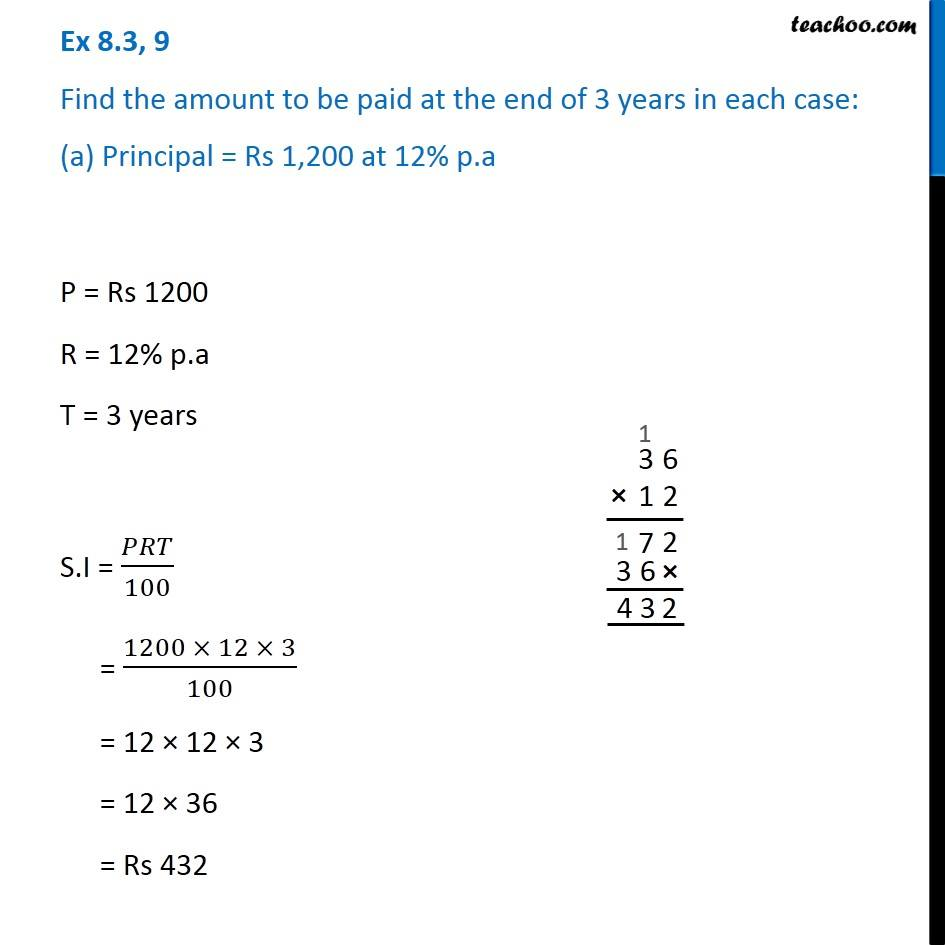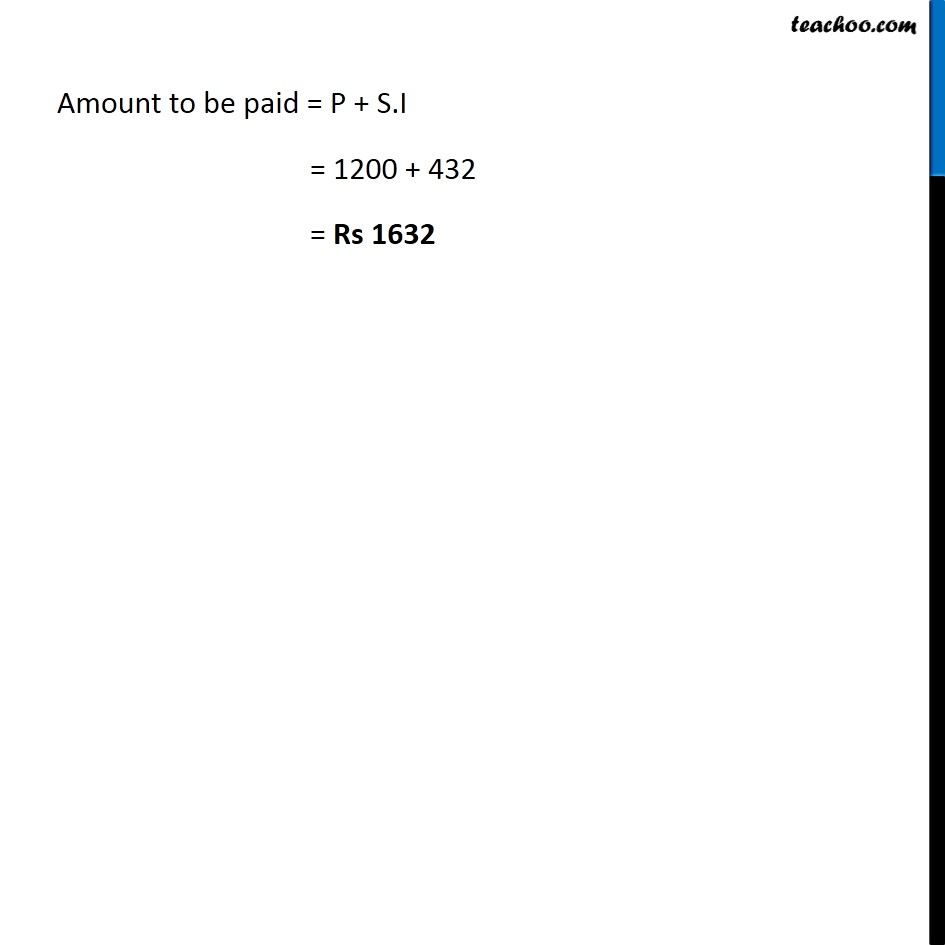Simple interest

Chapter 8 Class 7 Comparing Quantities
Concept wiseIntroducing your new favourite teacher - Teachoo Black, at only ₹83 per month

### Transcript

Ex 8.3, 9 Find the amount to be paid at the end of 3 years in each case: (a) Principal = Rs 1,200 at 12% p.a P = Rs 1200 R = 12% p.a T = 3 years S.I = 𝑃𝑅𝑇/100 = (1200 × 12 × 3)/100 = 12 × 12 × 3 = 12 × 36 = Rs 432 Amount to be paid = P + S.I = 1200 + 432 = Rs 1632